GeeksforGeeks App
Open AppBrowser
Continue

## Related Articles

Graphs are very necessary in the field of calculus, many times it is easier to do a lot of things or get an idea about the behavior of the function. The nature of any equation can easily be identified using graphs, for example, depending upon what the equation is, it can be answered whether the graph is a parabola, hyperbola, ellipse, etc. While sketching a Curve, there are various steps taken like finding the domain of the function, drawing the intercept, and so on.

### How to perform Curve Sketching?

For drawing the graph for any given function, there are a few steps and checks we need to follow. We need to check the derivatives, intersections, and also sometimes double derivatives to find out the behavior of the function. Sometimes functions are discontinuous, in that case, we will have to check all these things for each continuous piece. The list below indicates the things we need to check to get a fair idea of how the graph of any function would look like.

Domain

First, analyze the function to check for its domain. We need to find out the points where the value of the function becomes undefined or is discontinuous. For example:is not defined at x = 0. Log(x) is defined only at positive values of x.

Intercepts

Now after checking the domain, we will look for the points where the graph cuts the x-axis and the y-axis. This gives us a lot of idea about the shape of graphs. For finding out the x-intercept, just put y = 0 and solve the equation. Similarly, for finding out the values of the y-intercept, just put x = 0 and solve the reduced equation to get the value of y.

Symmetry

Determine whether the functions are odd, even, or neither of these. Sometimes some functions are periodic in nature. We need to check for their periodicity if they are periodic in nature. Functions satisfying, f(x) = f(-x) are called even functions. While the functions satisfying f(-x) = -f(x) are called odd functions. Some examples of periodic functions are:

Sin(x), Cos(x) and other trigonometric functions.

Maxima and Minima

Maxima and minima of the functions give us an idea about the regions where the function is increasing or decreasing. For finding out the maxima and minima in any function, we need to find the critical points. Critical points of the function are defined as the points where,

f'(x) = 0

Concavity and Convexity

Second derivative test must be used to find the points of inflection. Points of inflection occur when f”(x) = 0. The places where f”(x) < 0, that means the curve is convex upward while the places where f”(x) > 0, the curve is convex downward.

Asymptomatic Values

We also need to look the values, the function is going to take at x = ∞ or x = -∞. These values give idea about which way the graph of function will lie in the long term on larger values of x.

So, following these general rules, we can sketch graphs for any curve. Let’s look at the curves of polynomials and logarithmic functions.

### Sketching Polynomial Functions

Polynomial functions occur a lot in calculus, and it is essential to know how to sketch their graphs. We will look at a function and use the techniques studied above to infer the graph of the function. The general idea is to look for asymptomatic values, where they are going and then find the critical points and draw a graph according to them. Let’s see it through examples,

Example: Sketch the graph for the given function,

f(x) = x2 + 4

Solution:

We know that the domain of this function is all real numbers. This functions will tend to infinity as we go towards large positive and negative values of x.

Notice that f(-x) = (-x)2 + 4 = x2 + 4 = f(x). That is this function is even, so its graph must be symmetric about the y-axis.

Now we know that graph goes to infinity and is symmetrical around the y-axis. Now, let’s look for critical points.

f'(x) = 2x = 0

⇒ x = 0

Thus, there is only one critical point which is x = 0. Checking the double derivative f”(x) = 2. Since f”(x) > 0 for every x. So, the graph must be convex upward everywhere with minima at x = 0. Now we just need to know the value of the function at minima.

f(0) = 4.

Now we are ready to plot a graph.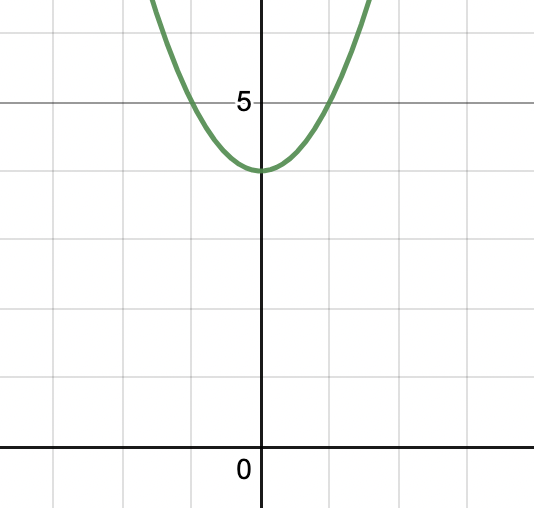### Sketching Logarithmic Functions

We know that logarithmic functions are inverse of exponential functions. The function y = logbx is the inverse of y = bx. The graph of the exponential function is given below. We also know that the graph of an inverse of a function is basically a mirror image of the graph in y = x. So we can derive the shape of the graph of log function from the given graph of the exponential function.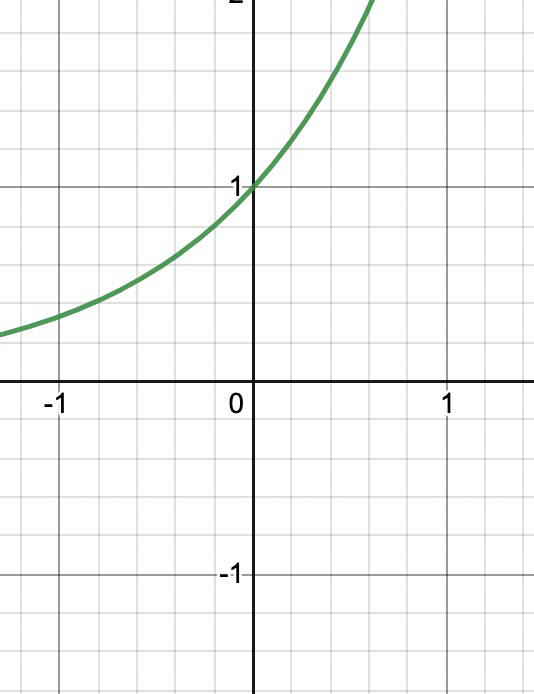The mirror image of this graph will be,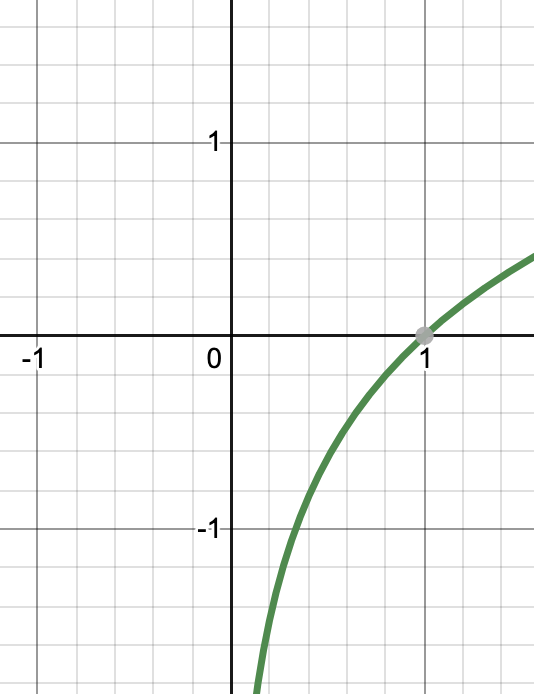Let’s see an example of graphic logarithmic functions.

Example: Plot the graph for log10x + 5.

Solution:

We can see that the function is f(x) = log10x + 5.

The graph of this equation will be shifted 5 units in the upwards direction.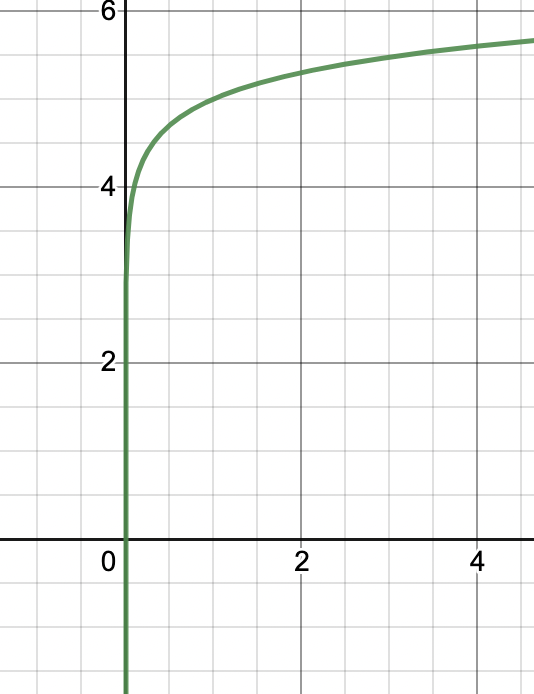### Sample Problems

Question 1: Sketch the graph for the given function,

f(x) = x + 8

Solution:

We know that the domain of this function is all real numbers. This functions will tend to infinity as we go towards large positive and negative values of x.

Now we know that graph goes to positive infinity for larger positive values of x and negative infinity for larger negative values of x.Now, let’s look for critical points.

f'(x) = 1

There is no critical point, that means derivatives change sign remains same and constant throughout.

Let’s see where the equation cuts the x-axis.

x+ 8 = 0

⇒x = -8

Now we are ready to plot a graph.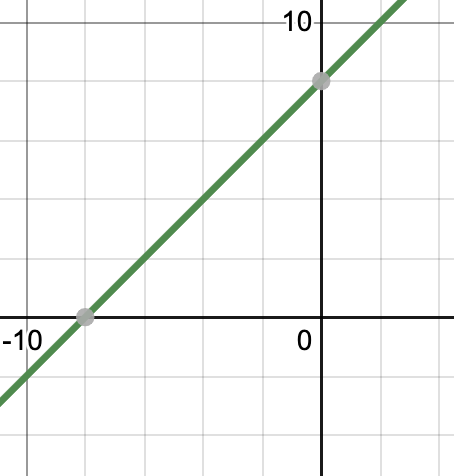Question 2: Sketch the graph for the given function,

f(x) = x2 – 6x + 8

Solution:

We know that the domain of this function is all real numbers. This functions will tend to infinity as we go towards large positive and negative values of x.

Now we know that graph goes to positive infinity for larger positive values of x and negative infinity for larger negative values of x.Now, let’s look for critical points.

f'(x) = 2x -6 = 0

⇒x = 3

There is one critical point, that means derivatives change sign at that, but we don’t know which sign changes to what. So, we will check the sign.

From x ∈ (-∞,3] f'(x) < 0. That is in this interval, the graph is decreasing.

From x ∈ (3,∞) f'(x) > 0. That is in this interval, the graph is increasing.

That means the critical point is a minimum.

Let’s see where the equation cuts the x-axis.

x2 -6x + 8 = 0

⇒x2 -4x -2x + 8 = 0

⇒x(x – 4) -2(x – 4) = 0

⇒(x – 2)(x – 4) = 0

Now we are ready to plot a graph.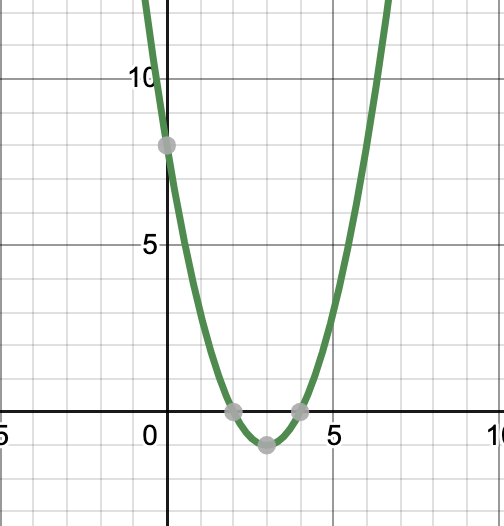Question 3: Sketch the graph for the given function,

f(x) = x3 – 3x + 4

Solution:

We know that the domain of this function is all real numbers. This functions will tend to infinity as we go towards large positive and negative values of x.

Now we know that graph goes to positive infinity for larger positive values of x and negative infinity for larger negative values of x.Now, let’s look for critical points.

f'(x) = 3x2 -3 = 0

⇒x2 = 1

⇒x = -1 or 1

There are two critical points, that means derivatives change sign at them, but we don’t know which sign changes to what. So, we will check the sign.

From x ∈ (-∞,-1] f'(x) > 0. That is in this interval, the graph is increasing.

From x ∈ (-1,1] f'(x) < 0. That is in this interval, the graph is decreasing.

From x ∈ (1,∞) f'(x) > 0. That is in this interval, the graph is increasing.

f(0) = 4.

Now we are ready to plot a graph.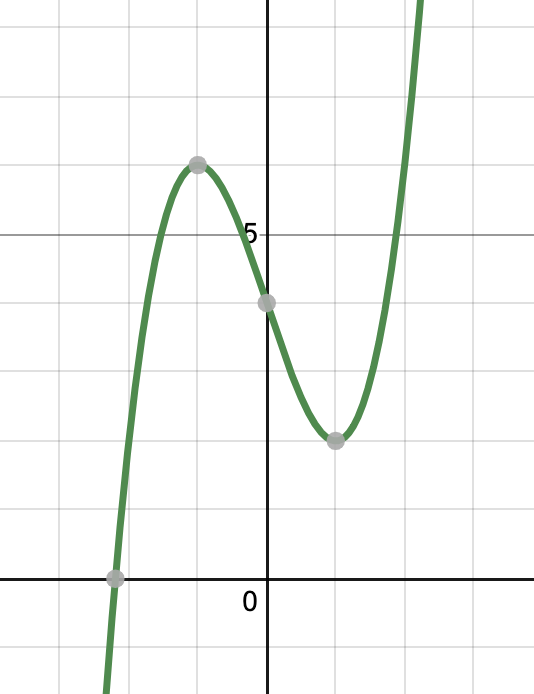Question 4: Find the graph for the equation f(x) = e + 2.

Solution:

We know that f(x) = ex + 2 is an exponential function, it increases with increasing value of x.

f'(x) = e

This will never become zero, so there are no critical points. The graph is continuously increasing.

f”(x) > 0 thus it’s shape is always convex upward. Due to the addition of 2 to the exponential function. The whole graph will be shifted two units upwards.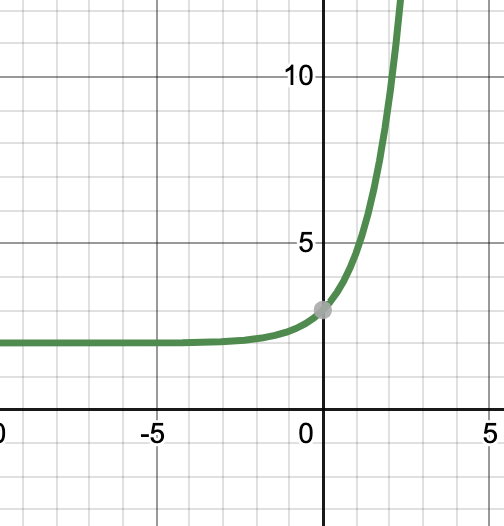My Personal Notes arrow_drop_up
Related Tutorials